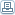# Differences Between Abacus Math and Vedic Math

 By: Dhaval ShrimankarToday two different ancient methods are available for school students to conquer math. With either of this technique students are able to do large and complex calculations in their head without need of any modern day electronic devices. These techniques are: 1) Abacus Math and 2) Vedic Math. Before we indulge straight into the differences let us first know what these two methods are.The abacus is an ancient tool invented during Greek and Roman times. Over the period abacus has evolved into different types as it traveled across the world. However it was in China the abacus was innovated further and used heavily in day-to-day life for calculations. Abacus math is a technique derived from this very old technique and fits right into the modern day world. Abacus use can build confidence, provide a sense of achievement, promote intuitive thinking, enhance problem-solving capability, stimulate creativity and improve concentration and mental endurance.Vedic Mathematics has no legal definition. Hindu philosophy is based on Veda-s (ancient scriptures written in Sanskrit, some say as old as 5000 B.C.) and mathematics translated from these texts is termed as Vedic Mathematics. These ancient scriptures were rediscovered by Sri Bharati Krsna Tirthaji (1884-1960) and he translated the complex text into simpler understandable mathematics.The system is based on 16 Vedic sutras or aphorisms, which are actually word-formulae describing natural ways of solving a whole range of mathematical problems. Some examples of sutras are "By one more than the one before", "All from 9 & the last from 10", and "Vertically & Crosswise". These 16 one-line formulae originally written in Sanskrit, which can be easily memorized, enables one to solve long mathematical problems quickly. These formulae describe the way the mind naturally works and are therefore a great help in directing the student to the appropriate method of solution.With Abacus math, abacus is used as a tool to learn calculations. Beads are moved up and down and various columns to represent the number. When children use both hands to move the abacus beads to perform arithmetic calculations, there is quick communication between the hands and the brain that stimulates both the right and left hemispheres of the brain. This promotes rapid, balanced whole brain development. Abacus math should be started at very early childhood, as young as age 4. Abacus math starts at very basic level by teaching the numbers and then progressing to calculations. Eventually the child retains the memory of bead positions and the relevant notation. Abacus math if started during later ages can create a bit of hindrance.Vedic math is entirely done in mind. Vedic math also starts at a basic level of numbers and gradually progressing to simple additions, subtractions, multiplications and division. Vedic math goes much more beyond just the basic calculations. With Vedic math one can also solve complex geometrical theorems and algebraic problems. Vedic math can be started at later ages as well without any difficulty.The key in both techniques is to practice and implement the methods in your day-to-day life. And what could be a better way to practice then using the math worksheets. Today there are various websites you can refer to gain knowledge of either method.